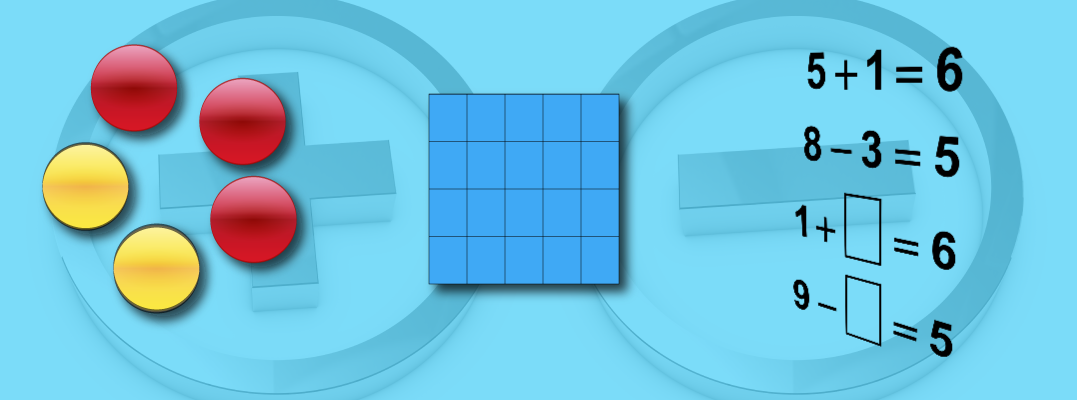# Unit: Addition and Subtraction 1### Key topics include:

• Basic Facts: Sums to 10
• Basic Facts: Sums to 20
• Basic Facts: Differences within 20
• Even and Odd Numbers

### The suggested timeframe for each lesson can be adapted to suit your needs.### Days 1-2: Speaking Math

Goal: Gain an understanding of the key words used throughout this unit.

These are the key terms you will learn in this unit:

2. Subtraction
3. Sum
4. Difference
6. Subtracting
7. Plus Sign
8. Minus Sign
9. Equals Sign
10. True Equation
11. False Equation
12. Commutative Property
13. Associative Property
14. Odd
15. Even

Day 1

• Key Terms
• Slide Show: Math Vocabulary
• Word Search Puzzles

Day 2

• Tutorial: Addition and Subtraction Equations
• Tutorial: Visual Models for Equations
• Writing Subtraction Equations

Media4Math Resources:### Days 3-4: Building Blocks

Goal: To develop a concrete understanding of addition and subtraction using various visual and tactile models.

Day 3

• Video Tutorial Reviews: Counting On and Skip-Counting
• Video Tutorial: Counting and Addition

Day 4

• Video Tutorial: Counting and Subtraction
• Subtracting with Number Lines

Media4Math Resources:### Days 5-6: Putting it Together

Goal: Use the Properties of Operations to add and subtract numbers. Develop a facility for basic facts.

Day 5

• Interactive Math Game: Add 'Em Up (Sums to 10)
• Interactive Math Game: Add 'Em Up (Sums to 20)
• Worksheet: Sums to 20

Day 6

• Tutorial: Subtraction Facts
• Interactive Math Game: What's the Diff?
• Worksheet: Differences to 10

Media4Math Resources:### Days 7-8: Show What You Know

Using addition and subtraction skills to explore even and odd numbers and solve simple equations.

Day 7

• Interactive Math Game: Whack a Turkey: Even Numbers
• Interactive Math Game: Whack a Turkey: Odd Numbers
• Tutorial: Properties of Even and Odd Numbers
• Worksheets: Even and Odd Numbers

Day 8

• Tutorial: Solving Equations